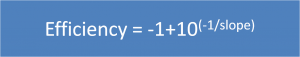# qPCR slope to efficiency

With this online tool you can calculate the % of efficiency of the PCR reaction, the exponent and the amplicication from the slope produced by the quantitative PCR standard curve.

Slope values between -3.1 and -3.6 are acceptable, and they will give reaction efficiencies between 90% and 110%.

The formula to calculate the efficiency is the following: